## Introduction to Clojure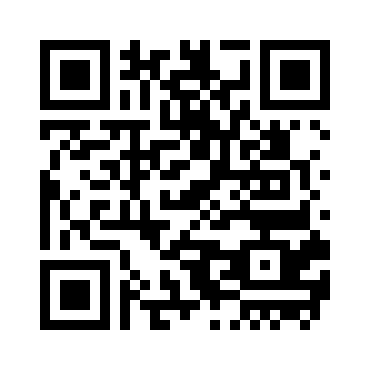## Tools## Topics

 Functions Lists Vectors Maps Sets## Functions

A function is an entity that receives "things" and return a "thing".The beauty of functional programming is that the "things" that functions receive and return can be anything - including functions

In functional programming, functions are first-class citizens.

## Functions

There are 3 ways to define a function and one way to call a function:

1. With `fn`:
``````(def foo (fn [a b c] a))
(foo 1 2 3)``````
2. With the anonymous function literal: `#`:
``(#(+ %1 %3) 1 10 100)``
3. With `defn`, you can add a documentation string to your function:
`````` (defn bar "bar is a function with documentation"
[a b c] b)
(bar 1 2 3)``````

## Functions - (defn bar) vs. (def bar fn)

`defn` is a macro that associates metadata to the function that it defines:
`````` (defn bar "bar is a function with documentation"
[a b c] b)
(meta #'bar)``````
For instance, we can retrieve the documentation string of a function at run time:
`` (:doc (meta #'inc)) ``

## Functions - Practice

Write a function that receives a name and returns a string with the concatanation of "Hello" and the name. You'll need to use the str function.
`` (defn hello [name]) ``
Write a function that receives a number and returns "zero" if the number is zero and "non-zero" otherwise. Take a look at if.
`` (defn zero-check [n]) ``

Write a function called `applicator` that receives two arguments: a function and a "thing" and returns the application of the function on the argument.

`````` (defn applicator [func number])
(= (applicator inc 19) 20) ``````

## Lists

In clojure, `Lists` are simply linked lists.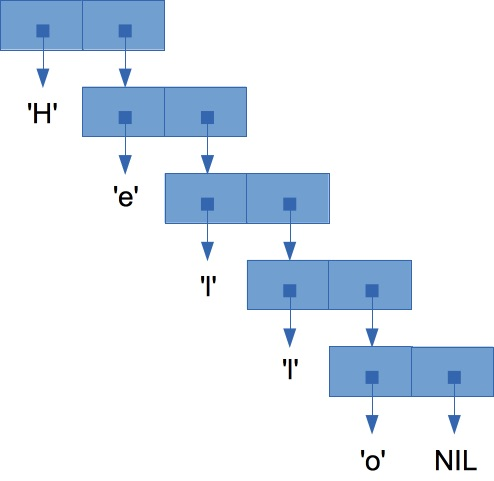## List Creation

You can mix element of different types inside a list:
`` (list 1 2 "hello") ``
You can nest lists inside lists inside lists inside lists...
`` (list 1 2 (list "a" "b") (list 1.2 (list 3.43243 2.5))) ``
There is a kind of a syntactic sugar for list creation: the single quote `'`.
And it works fine with nested lists:
``'(1 2 ("a" "b" (1 2 4)))``

## Functions on lists (List API)

The most natural manipulation on a list is to take the list without its first element:
``(rest '(1 2 3 4 5))``
In order to get the first element of a list, use `first`:
``(first '(1 2 3 4 5))``
Lists are linked lists, therefore elements are prepended to the head of the list:
``(cons 1 '(2 3))``
Like all the clojure collections, you can `count` the elements of a list:
``(count '(1 2 3 4 5))``

## Lists - Practice

Write a function that receives a list and returns its fifth element. You'll need to use the rest function a couple of times.
`````` (defn fifth [lst])
(fifth '(1 2 3 4 5 6 7 8)) ``````

Write a function that receives a number `n` and a list and returns its `n`th element. You'll need to use recursion.

`````` (defn nth-element [lst n])
(nth-element '(1 2 3 4 5 6 7 8 9 10 11) 10) ``````

Write a function called `select-1-5-7` that receives a list and returns a list with the elements of the original list at position 1 5 and 7.

You might use either your `nth-element` or the clojure nth.
`` (defn select-1-5-7 [lst]) ``

## Vectors

`Vectors` are like `Lists` but with fast random access.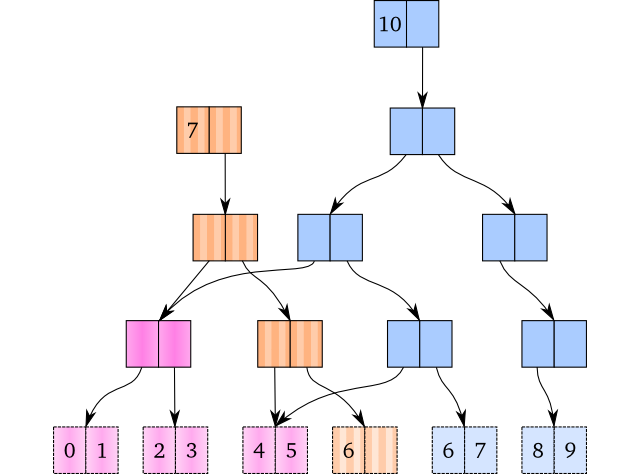## Vector Creation

Vectors are created using `vector`
`` (vector 1 2 3 4 5) ``
Vectors can also be created using the literal `[]`
`` [1 2 3 4 "aa" [1 2] [1 2 3]] ``
Like with `Lists`, you can access the first element with `first`:
`` (first [1 2 3 4 5]) ``
And you can also access any element in the vector and it is efficient:
`` (get [0 1 2 3 4 5 6 7 8 9 10] 7) ``
You can convert a `list` into a `vector` with `vec`:
``(vec '(1 2 3 4 5))``

## Vectors - Practice

Write a function that receives a vector and converts it to a list:
`` (defn ->list [vec]) ``
Write a function that receives a "thing" and a vector and returns true or false according to the presence of the "thing" in the vector:
`` (defn present? [vec thing]) ``

## Maps

Maps are associative arrays (sometimes called dictionaries).
Basically maps are key-value based collections.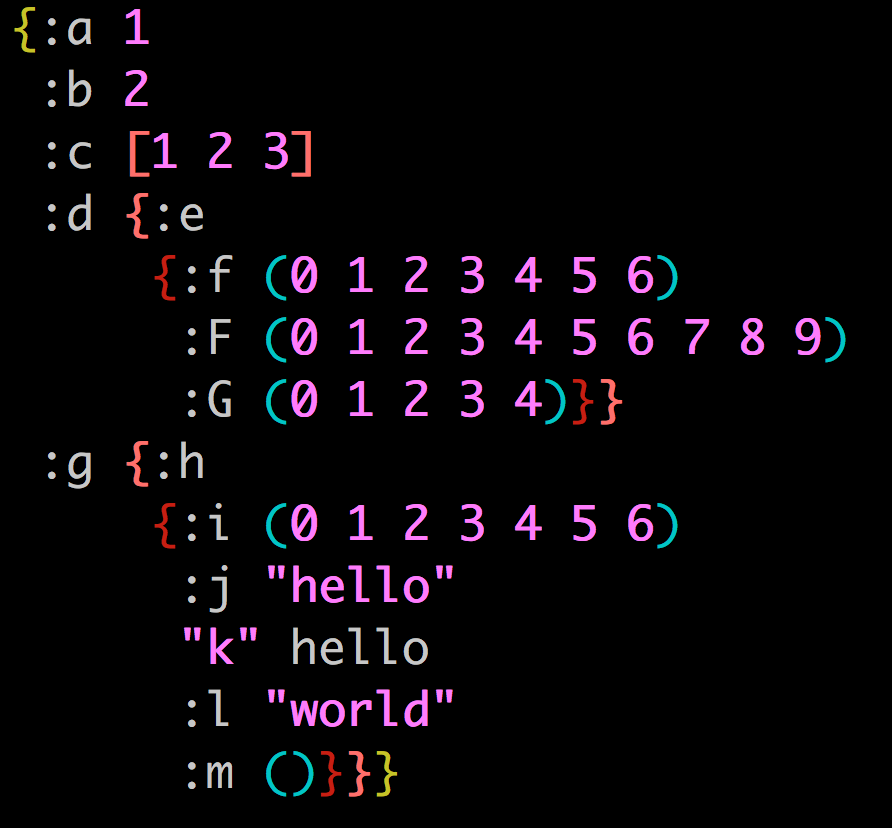## Maps - creation

There are a couple of ways to create a map:

1. With `hash-map`:

``(hash-map :a 2 :b 3 :c 4 :d 5 :e 6)``
2. With the map brackets literals `{}`:

``{:a 1 :b "aa" :c 'a}``

Pay attention that there is no meaning to the order of the elements inside a map.

Also, if you set a key twice, one will override the other.

Maps can be nested:

``{:x {:a 1 :b "aa" :c 'a} :y [1 2 {:a 3}]}``

Keys and values can be of any types: lists, vectors, keywords, regexps and even maps and functions!

``````'{:email #"^([a-zA-Z0-9_\-\.]+)@([a-zA-Z0-9_\-\.]+)\.([a-zA-Z]{2,5})\$"
(1 2 3 4) list
[1 2 3] vector
{:d 1} map}``````

## Maps - creation (cont.)

1. With `zipmap`, you set the keys and the values separately:

``(zipmap (range 10) (range 100))``
2. With `(into {})`, you can convert a `2d` array into a map:

``(into {} [[:a 2] [:b 3] [:c 4] [:d 5] [:e 6]])``

What methods don't support nested maps creations?

How many keys are there in this map: `{[1 2] :aaa '(1 2) :aaa}`?

How do you generate an empty map?

## Maps - functions

With `assoc`, you associate (create or modify) one or more `key-value`s

``(assoc {} :a 1 :b 2)``

With `dissoc` you dissociate (remove) one or more `key-value`s

``(dissoc {:a 1 :b 2} :a :b)``

With `assoc-in`, you associate a nested `key-value`

``(assoc-in {} [:a :b :c] 1)``

What will happen with `(assoc-in {:a 1} [:a :b :c] 1)`?

## Maps - functions (cont.)

There are a couple of ways to retrieve values from a map:

1. get

``(get {:a 1 :b 2} :a)``

When they key is not there, `get` returns `nil`:

``(get {} :a)``

Meditate about this problematic code: `(get {:a nil} :a)`

What happens if you pass an additional argument to `get`?

2. get-in

``(get-in {:a {:b 1}} [:a :b])``
3. find

``(find {:a 1 :b 2} :a)``

## Maps - functions (cont.)

1. the map itself is a function

``({:a 1} :a)``
2. keywords are functions

``(:a {:a 1})``

You can retrieve the keys of the map with `keys`

``(keys {:a 1 :b 2 :c {:d 1}})``

And the values with `vals`

``(vals {:a 1 :b 2 :c {:d 1}})``

Write an assertion using `keys`, `vals` and `zipmap`.

## Maps - Practice

1. Write a function that returns the values for a selected collection of keys

``````(defn my-select-keys [m the-keys])
(= (my-select-keys {:a 1 :b 2}) [:a :b :c] '(1 2 nil))``````
2. Write a function that receives a map `m` and a function `f` and returns a map with the same keys as `m` and with the values transformed by `f`:

``````(defn map-object [f m])
(= (map-object #(* 100 %) {:a 1 :b 2 :c 3}) {:a 100 :b 200 :c 300})``````
3. Write a function that converts a sequence into a map where the keys are the indexes of the elements in the sequence.

``````(defn sequence->map [s])
(= (sequence->map [10 20 30]) {0 10 1 20 2 30})``````
4. Write a function that checks if a map is a submap of the other.
Map m1 is a submap of m2 if all key/value pairs in m1 exist in m2"

``````(defn submap [m1 m2])
[(true? (submap {:a 1 :b 2} {:a 1 :b 2 :c 3}))
(false? (submap {:a 1 :b 2 :c nil} {:a 1 :b 2}))]``````

## Sets

Set is a fundamental concept of mathematics.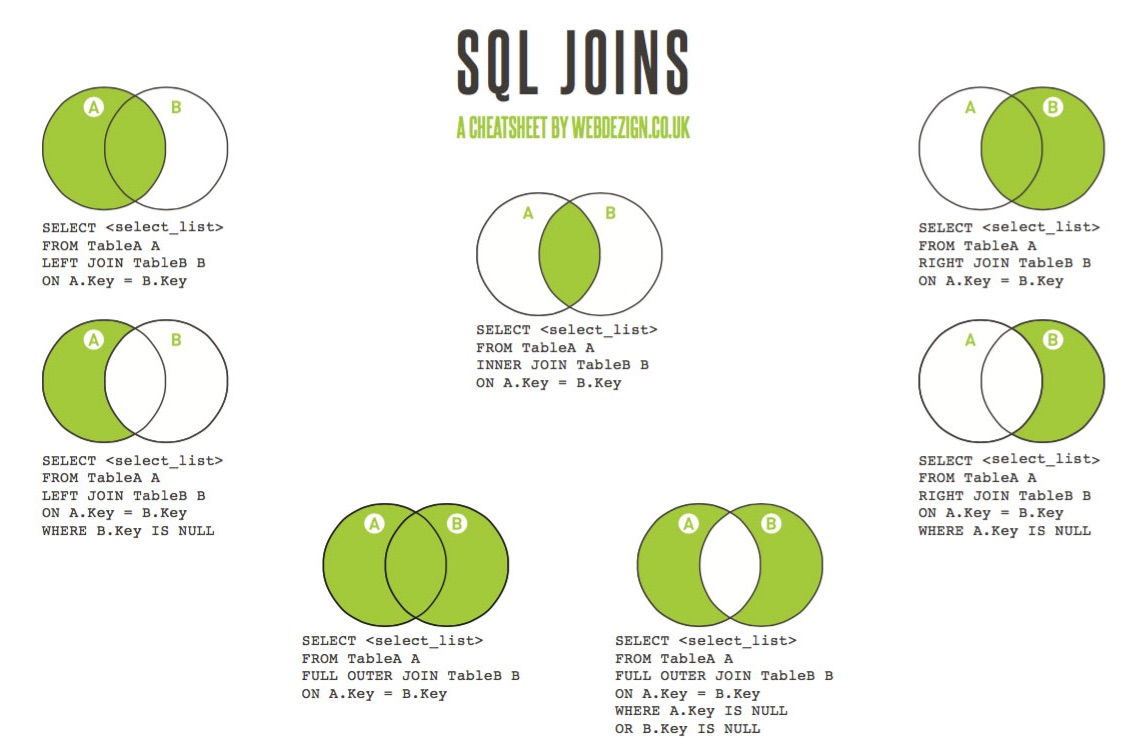## Sets - creation

To create a `set` from a collection, you use `set`:

``(set '(1 "hello" [1 2]))``

In a set, there are no duplicates

``(set [1 1 "hello" "hello" [1 2] '(1 2)])``

Why `[1 2]` and `(1 2)` are considered as duplicates?

You can also create a set with the set literal `#{}`

``#{1 2 "hello" [1 2]}``

But then, duplicates are forbidden!

``#{1 2 3 1}``

What's the difference between `set` and `distinct`?

``(distinct [1 1 "hello" "hello" [1 2] '(1 2)])``

You can also use `(into #{})` to create a set

``(into #{} [1 2 3 3 4])``

## Sets - functions

In order to use the functions on sets, we have to require the `clojure.set` namespace:

``(require '[clojure.set :as s])``

Why is there a quote in the `require` statement?

All the basic mathematical operations on sets are available:

1. Union

``(s/union #{1 2} #{2 3} #{4 5 2})``
2. Intersection

``(s/intersection #{1 2} #{2 3})``
3. Difference

``(s/difference #{1 2 3 4 5} #{1 2} #{3 4})``You are on your way to become a `clojure` expert: